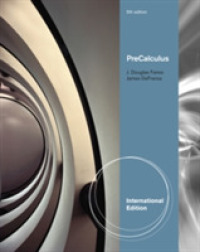Precalculus, International Edition -- Paperback （5 ed）

• ただいまウェブストアではご注文を受け付けておりません。 ⇒古書を探す
• 製本 Paperback:紙装版/ペーパーバック版
• 商品コード 9780840068880

Full Description

Make the grade with PRECALCULUS, 5e, International Edition and its accompanying technology! With a focus on teaching the essentials, this streamlined mathematics text provides you with the fundamentals necessary to be successful in this course-and your future calculus course. Exercises and examples are presented in the same way that you will encounter them in calculus, familiarizing you with concepts you'll use again, and preparing you to succeed. In-text study aids further help you master concepts.

Contents

Chapter 1 Functions.1.1 Introduction. 1.2 The Real Line. 1.3 The Coordinate Plane.1.4 Equations and Graphs.1.5 Using Technology to Graph Functions.1.6 Functions.1.7 Linear Functions. 1.8 Quadratic Functions.Review Exercises.Exercises for Calculus. Chapter Test.Chapter 2 New Functions From Old.2.1 Introduction.2.2 Other Common Functions.2.3 Arithmetic Combinations of Functions. 2.4 Composition of Functions. 2.5 Inverse Functions.Review Exercises.Exercises for Calculus. Chapter Test.Chapter 3 Algebraic Functions. 3.1 Introduction.3.2 Polynomial Functions. 3.3 Finding Factors and Zeros of Polynomials. 3.4 Rational Functions. 3.5 Other Algebraic Functions.3.6 Complex Roots of Polynomials.Review Exercises.Exercises for Calculus. Chapter Test.Chapter 4 Trigonometric Functions. 4.1 Introduction. 4.2 Measuring Angles. 4.3 Right-Triangle Trigonometry. 4.4 The Sine and Cosine Functions. 4.5 Graphs of the Since and Cosine Functions. 4.6 Other Trigonometric Functions. 4.7 Trigonometric Identities. 4.8 Inverse Trigonometric Functions. 4.9 Additional Trigonometric Applications. Review Exercises. Exercises for Calculus. Chapter Test. Chapter 5 Exponential and Logarithm Functions.5.1 Introduction.5.2 The Natural Exponential Function.5.3 Logarithm Functions.5.4 Exponential Growth and Decay.Review Exercises. Exercises for Calculus. Chapter Test.Chapter 6 Conic Sections, Polar Coordinates, and Parametric Equations.6.1 Introduction. 6.2 Parabolas. 6.3 Ellipses.6.4 Hyperbolas.6.5 Polar Coordinates.6.6 Conic Sections in Polar Coordinates.6.7 Parametric Equations.Review Exercises.Exercises for Calculus .Chapter Test.